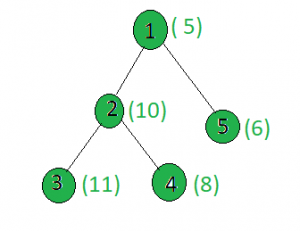# Count the nodes in the given tree whose weight is prime

Given a tree, and the weights of all the nodes, the task is to count the number of nodes whose weight is prime.

Examples:

Input:Output: 2
Only the weights of the nodes 1 and 3 are prime.

## Recommended: Please try your approach on {IDE} first, before moving on to the solution.

Approach: Perform dfs on the tree and for every node, check if it’s weight is prime or not.

Below is the implementation of above approach:

## C++

 `// C++ implementation of the approach ` `#include ` `using` `namespace` `std; ` ` `  `int` `ans = 0; ` ` `  `vector<``int``> graph; ` `vector<``int``> weight(100); ` ` `  `// Function that returns true ` `// if n is prime ` `bool` `isprime(``int` `n) ` `{ ` `    ``for` `(``int` `i = 2; i * i <= n; i++) ` `        ``if` `(n % i == 0) ` `            ``return` `false``; ` `    ``return` `true``; ` `} ` ` `  `// Function to perform dfs ` `void` `dfs(``int` `node, ``int` `parent) ` `{ ` `    ``// If weight of node is prime or not ` `    ``if` `(isprime(weight[node])) ` `        ``ans += 1; ` ` `  `    ``for` `(``int` `to : graph[node]) { ` `        ``if` `(to == parent) ` `            ``continue``; ` `        ``dfs(to, node); ` `    ``} ` `} ` ` `  `// Driver code ` `int` `main() ` `{ ` `    ``// Weights of the node ` `    ``weight = 5; ` `    ``weight = 10; ` `    ``weight = 11; ` `    ``weight = 8; ` `    ``weight = 6; ` ` `  `    ``// Edges of the tree ` `    ``graph.push_back(2); ` `    ``graph.push_back(3); ` `    ``graph.push_back(4); ` `    ``graph.push_back(5); ` ` `  `    ``dfs(1, 1); ` ` `  `    ``cout << ans; ` ` `  `    ``return` `0; ` `} `

## Java

 `// Java implementation of the approach ` `import` `java.util.*; ` ` `  `class` `GFG{ ` `  `  `static` `int` `ans = ``0``; ` ` `  `static` `Vector[] graph = ``new` `Vector[``100``];  ` `static` `int``[] weight = ``new` `int``[``100``]; ` `  `  `// Function that returns true ` `// if n is prime ` `static` `boolean` `isprime(``int` `n) ` `{ ` `    ``for` `(``int` `i = ``2``; i * i <= n; i++) ` `        ``if` `(n % i == ``0``) ` `            ``return` `false``; ` `    ``return` `true``; ` `} ` `  `  `// Function to perform dfs ` `static` `void` `dfs(``int` `node, ``int` `parent) ` `{ ` `    ``// If weight of node is prime or not ` `    ``if` `(isprime(weight[node])) ` `        ``ans += ``1``; ` `  `  `    ``for` `(``int` `to : graph[node]) { ` `        ``if` `(to == parent) ` `            ``continue``; ` `        ``dfs(to, node); ` `    ``} ` `} ` `  `  `// Driver code ` `public` `static` `void` `main(String[] args) ` `{ ` `    ``for` `(``int` `i = ``0``; i < ``100``; i++)  ` `        ``graph[i] = ``new` `Vector<>(); ` `     `  `    ``// Weights of the node ` `    ``weight[``1``] = ``5``; ` `    ``weight[``2``] = ``10``; ` `    ``weight[``3``] = ``11``; ` `    ``weight[``4``] = ``8``; ` `    ``weight[``5``] = ``6``; ` `  `  `    ``// Edges of the tree ` `    ``graph[``1``].add(``2``); ` `    ``graph[``2``].add(``3``); ` `    ``graph[``2``].add(``4``); ` `    ``graph[``1``].add(``5``); ` `  `  `    ``dfs(``1``, ``1``); ` `  `  `    ``System.out.print(ans);  ` `} ` `} ` ` `  `// This code is contributed by Rajput-Ji `

## Python3

 `# Python3 implementation of the approach ` `ans ``=` `0` ` `  `graph ``=` `[[] ``for` `i ``in` `range``(``100``)] ` `weight ``=` `[``0``] ``*` `100` ` `  `# Function that returns true ` `# if n is prime ` `def` `isprime(n): ` `    ``i ``=` `2` `    ``while``(i ``*` `i <``=` `n): ` `        ``if` `(n ``%` `i ``=``=` `0``): ` `            ``return` `False` `        ``i ``+``=` `1` `    ``return` `True` ` `  `# Function to perform dfs ` `def` `dfs(node, parent): ` `    ``global` `ans ` `     `  `    ``# If weight of the current node is even ` `    ``if` `(isprime(weight[node])): ` `        ``ans ``+``=` `1``; ` `     `  `    ``for` `to ``in` `graph[node]: ` `        ``if` `(to ``=``=` `parent): ` `            ``continue` `        ``dfs(to, node) ` ` `  `# Driver code ` ` `  `# Weights of the node ` `weight[``1``] ``=` `5` `weight[``2``] ``=` `10` `weight[``3``] ``=` `11` `weight[``4``] ``=` `8` `weight[``5``] ``=` `6` ` `  `# Edges of the tree ` `graph[``1``].append(``2``) ` `graph[``2``].append(``3``) ` `graph[``2``].append(``4``) ` `graph[``1``].append(``5``) ` ` `  `dfs(``1``, ``1``) ` `print``(ans) ` ` `  `# This code is contributed by SHUBHAMSINGH10 `

Output:

```2
```

Complexity Analysis:

• Time Complexity: O(N*sqrt(V)), where V is the maximum weight of a node in the given tree.
In DFS, every node of the tree is processed once and hence the complexity due to the DFS is O(N) when there are N total nodes in the tree. Also, while processing every node, in order to check if the node value is prime or not, a loop up to sqrt(V) is being run, where V is the weight of the node. Hence for every node, there is an added complexity of O(sqrt(V)). Therefore, the time complexity is O(N*sqrt(V)).
• Auxiliary Space: O(1).
Any extra space is not required, so the space complexity is constant.

Attention reader! Don’t stop learning now. Get hold of all the important DSA concepts with the DSA Self Paced Course at a student-friendly price and become industry ready.

My Personal Notes arrow_drop_upCheck out this Author's contributed articles.

If you like GeeksforGeeks and would like to contribute, you can also write an article using contribute.geeksforgeeks.org or mail your article to contribute@geeksforgeeks.org. See your article appearing on the GeeksforGeeks main page and help other Geeks.

Please Improve this article if you find anything incorrect by clicking on the "Improve Article" button below.